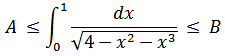# Interval of the Riemann integral value

• MHB
goody1
Hello everyone, I have to find an interval of this Riemann integral. Does anybody know the easiest way how to do it? I think we need to do something with denominator, enlarge it somehow. My another guess is the integral is always larger than 0 (A=0) because the whole function is still larger than 0 on interval from 0 to 1. Thank you in advance.

#### Attachments

•integral.png
1 KB · Views: 13

The function $\tfrac1{\sqrt{4-x^2-x^3}}$ increases on the interval $0\leqslant x\leqslant 1$. The minimum value occurs when $x=0$, and the maximum at $x=1$. You can use that to get estimates for $A$ and $B$.